### IMO Shortlist 2006 problem G5

Kvaliteta:
Avg: 3,0
Težina:
Avg: 8,0
In triangle$ABC$, let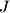$J$ be the center of the excircle tangent to side$BC$ at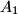$A_{1}$ and to the extensions of the sides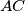$AC$ and$AB$ at$B_{1}$ and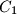$C_{1}$ respectively. Suppose that the lines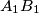$A_{1}B_{1}$ and$AB$ are perpendicular and intersect at$D$. Let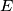$E$ be the foot of the perpendicular from$C_{1}$ to line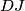$DJ$. Determine the angles$\angle{BEA_{1}}$ and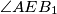$\angle{AEB_{1}}$.
Izvor: Međunarodna matematička olimpijada, shortlist 2006